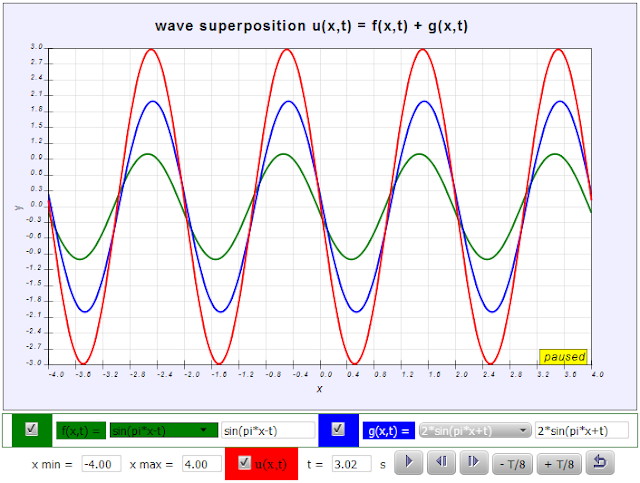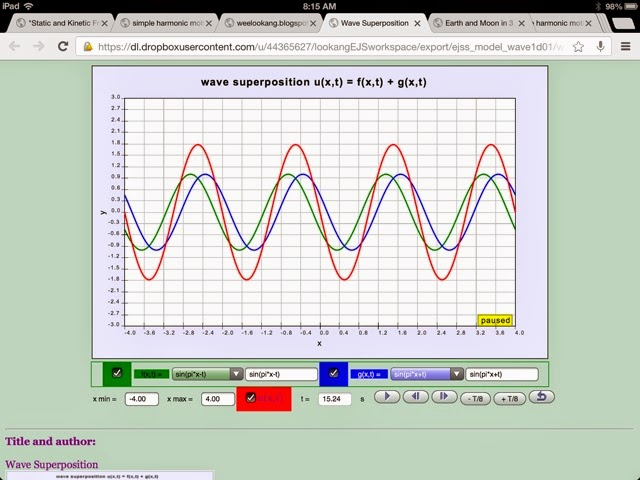# EJSS wave 1d superposition model

EJSS wave 1d superposition model by wolfgang christian and customized by lookang.

the following were reference for the customized of this model.

1. wolfgang and lookang  http://weelookang.blogspot.com/2010/06/open-source-ejs-superposition-of-2.html
2. One Dimensional Wave Superposition JS Model by Wolfgang Christian and Francisco Esquembre http://www.compadre.org/osp/items/detail.cfm?ID=13014http://weelookang.blogspot.sg/2013/12/ejss-wave-1d-superposition-model.html https://dl.dropboxusercontent.com/u/44365627/lookangEJSworkspace/export/ejss_model_wave1d01/wave1d01_Simulation.html source: https://dl.dropboxusercontent.com/u/44365627/lookangEJSworkspace/export/ejss_src_wave1d01.zip author:  Wolfgang and lookang (this version)

## Description:

The EJSS wave 1d superposition model  shows how the superposition principle gives rise to wave phenomena such as standing waves and beats. Users enter real-valued wave functions and dropdown menu for ease of inputs  and observe both the time dependent functions and their superposition. This model uses the JavaScript mathematical function parser.
A wave is a disturbance, such as sound, that propagates through space. For a wave propagating in one dimension, we use a wave function u(x,t) to represent the wave at position x and time t as shown in the screen shot.  Although a sinusoidal wave function is a very common type type of disturbance, we should remember that there are many other wave functions, such as shock waves, that do not fit this functional form.

The EJSS wave 1d superposition model  was developed using the Easy Java Simulations (EJS) version 5. It is distributed as a ready-to-run html page and requires only a browser with JavaScript support.

The mathematical equation used are:

f(x,t)=sin(πxt)

g(x,t)=sin(πx+t)

which the resultant is

u(x,t)=sin(πxt)+sin(πx+t)

the dropdown menu has some function typical in the A level Physics

f(x,t)=sin(πxt)
f(x,t)=12sin(πxt)
f(x,t)=2sin(πxt)
f(x,t)=12sin(πxt)
f(x,t)=2sin(πxt)
f(x,t)=sin(πx12t)
f(x,t)=sin(πx2t)
f(x,t)=sin(πxtπ2)

["sin(pi*x-t)","0.5*sin(pi*x-t)","2*sin(pi*x-t)","sin(0.5*pi*x-t)","sin(2*pi*x-t)","sin(pi*x-0.5*t)","sin(pi*x-2*t)","sin(pi*x-t-0.785)","sin(pi*x-t-1.57)"]

g(x,t)=sin(πx+t)
g(x,t)=12sin(πx+t)
g(x,t)=2sin(πx+t)
g(x,t)=12sin(πx+t)
g(x,t)=2sin(πx+t)
g(x,t)=sin(πx+12t)
g(x,t)=sin(πx+2t)
g(x,t)=sin(πxt+π2)

["sin(pi*x+t)","0.5*sin(pi*x+t)","2*sin(pi*x+t)","sin(0.5*pi*x+t)","sin(2*pi*x+t)","sin(pi*x+0.5*t)","sin(pi*x+2*t)","sin(pi*x-t+0.785)","sin(pi*x-t+1.57)"]

## in general:

f(x,t)+g(x,t)=u(x,t)

## Changes:

1. recreate on a fresh ejss using codes from http://www.compadre.org/osp/items/detail.cfm?ID=13014
2. use design ideas from http://weelookang.blogspot.com/2010/06/open-source-ejs-superposition-of-2.html
3. fix a bug in the input fields need _view._update(); //critical for immediate update

## Area of improvement: Done!

1. cannot figure out how to make a dot that travels on the  f(x,t) and  g(x,t)
the generic wave form has an equation of this form:

f(x,t)=sin(πxk1t) wave moves to the right

g(x,t)=sin(πx+k2t) wave moves to the leftPosted by
Labels: ejss WAVES